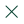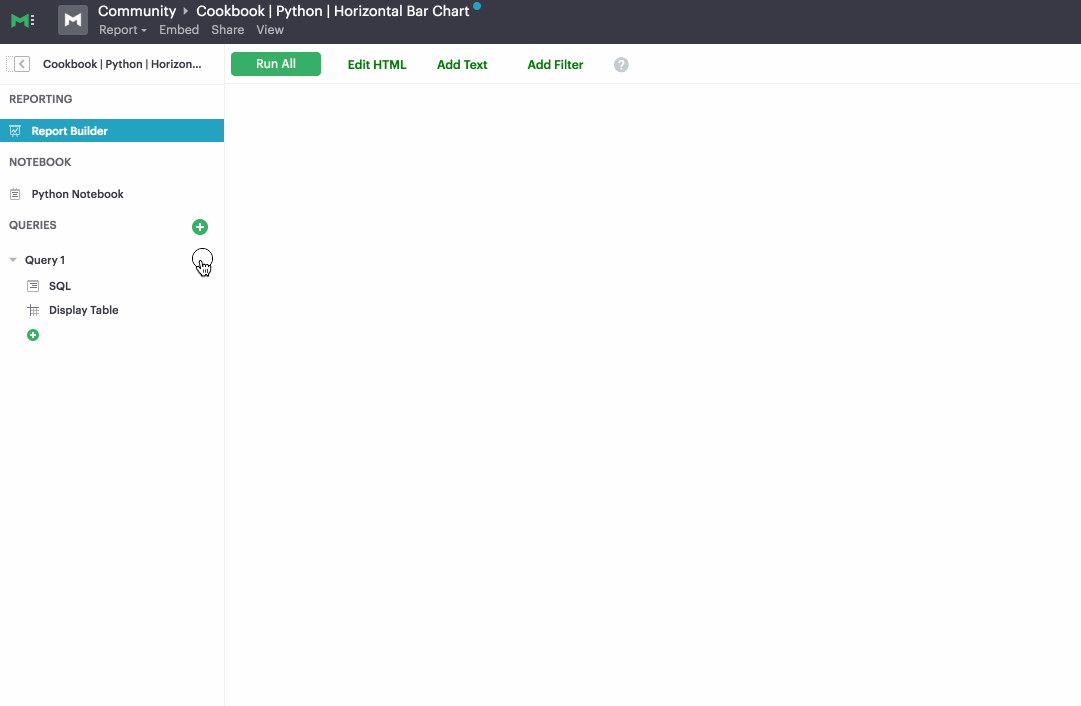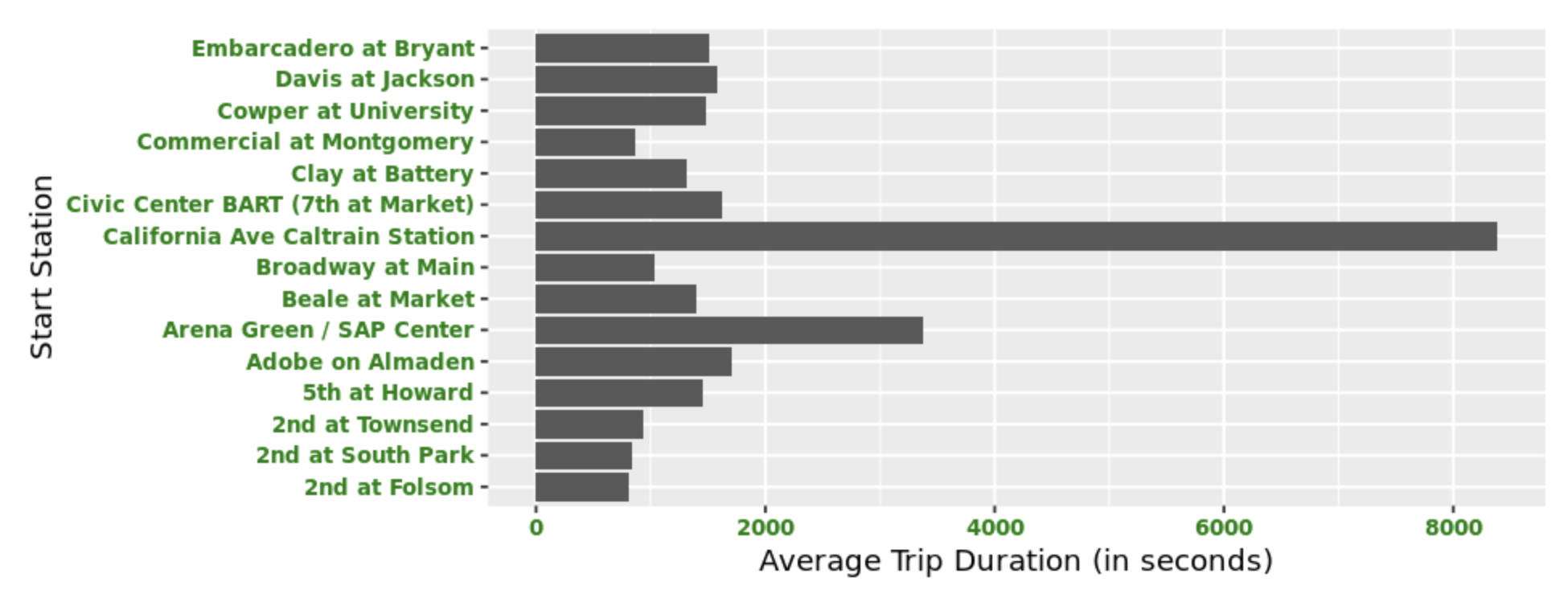ThoughtSpot acquires Mode to define the next generation of collaborative BI >>Learn More# Creating Horizontal Bar Charts using R

Often when visualizing data using a bar chart, you’ll have to make a decision about the orientation of your bars. While there are no concrete rules, there are quite a few factors that can go into making this decision. For example, when grouping your data by an ordinal variable, you may want to display those groupings along the x-axis. On the other hand, when grouping your data by a nominal variable, or a variable that has long labels, you may want to display those groupings horizontally to aid in readability.

This recipe will show you how to go about creating a horizontal bar chart using R. Specifically, you’ll be using the ggplot2 plotting system.

In our example, you'll be using the publicly available San Francisco bike share trip dataset to identify the top 15 bike stations with the highest average trip durations. You will then visualize these average trip durations using a horizontal bar chart. The steps in this recipe are divided into the following sections:

You can find implementations of all of the steps outlined below in this example Mode report. Let’s get started.

### Data Wrangling

You’ll use SQL to wrangle the data you’ll need for our analysis. For this example, you’ll be using the `sf_bike_share_trips` dataset available in Mode's Public Data Warehouse. Using the schema browser within the editor, make sure your data source is set to the Mode Public Warehouse data source and run the following query to wrangle your data:

```````select *
from modeanalytics.sf_bike_share_trips
`
``````

Once the SQL query has completed running, rename your SQL query to `SF Bike Share Trip Rankings` so that you can easily identify it within the R notebook:### Data Analysis

Now that you have your data wrangled, you’re ready to move over to the R notebook to prepare your data for visualization. Inside of the R notebook, start by importing the R libraries that you'll be using throughout the remainder of this recipe:

``````library(ggplot2)
library(magrittr)
library(dplyr)
``````

Mode automatically pipes the results of your SQL queries into an R dataframe assigned to the variable `datasets`. You can use the following line of R to access the results of your SQL query as a dataframe and assign them to a new variable:

```````bike <- datasets[['SF Bike Share Trip Data']]`
``````

As previously mentioned, your goal is to visualize the 15 start stations with the highest average trip duration. You can analyze the dataframe to find these stations using the following R code on our existing dataframe object:

``````#Grouping data by start_station_name
bike2 <- bike %>% group_by(start_station_name)
x <- bike2 %>% summarise(
duration = mean(duration)
)

``````

We now have a new dataframe assigned to the variable `y` that contains the top 15 start stations with the highest average trip durations. Now that we have our dataset aggregated, we are ready to visualize the data.

### Data Visualization

To create a horizontal bar chart, you can use the following snippet of R code, which utilizes the ggplot2 library:

``````options(repr.plot.width=8, repr.plot.height=3)
ggplot(y, aes(x = start_station_name, y = duration, main="Car Distribution")) +
geom_bar(stat = "identity") +
coord_flip() + scale_y_continuous(name="Average Trip Duration (in seconds)") +
scale_x_discrete(name="Start Station") +
theme(axis.text.x = element_text(face="bold", color="#008000",
size=8, angle=0),
axis.text.y = element_text(face="bold", color="#008000",
size=8, angle=0))
``````

which returns the following visualization: